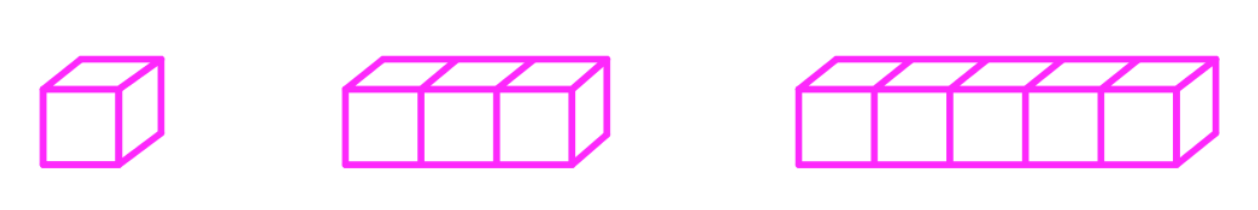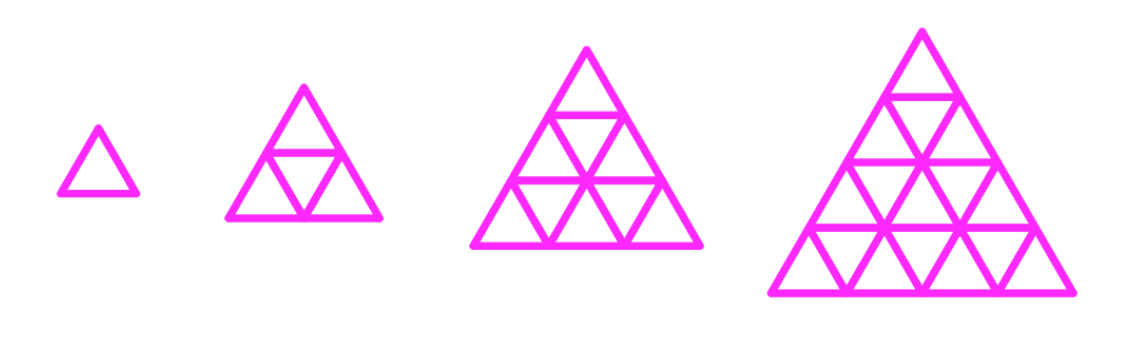# Inductive reasoning#### Everything You Need in One Place

Homework problems? Exam preparation? Trying to grasp a concept or just brushing up the basics? Our extensive help & practice library have got you covered.#### Learn and Practice With Ease

Our proven video lessons ease you through problems quickly, and you get tonnes of friendly practice on questions that trip students up on tests and finals.#### Instant and Unlimited Help

Our personalized learning platform enables you to instantly find the exact walkthrough to your specific type of question. Activate unlimited help now!##### Intros
###### Lessons
1. Inductive Reasoning Overview:
What are conjectures?
##### Examples
###### Lessons
1. Making a Conjecture

Make a conjecture of the next item or number based on the information given:

1. 1, 3, 5, 7, 9,…
2. $\frac{1}{2}, \frac{1}{3}, \frac{1}{4}, \frac{1}{5}$
3.4.5.6.2. Make a conjecture with the given information. Draw a figure to show that your conjecture is correct:
1. ABC is a triangle and AB = BC
2. ABC is a triangle and $\angle$B is a right angle.
3. Line $a$ and line $b$ are perpendicular
4. Line $a$ and line $b$ are parallel
3. Counterexamples of Conjectures
Determine if the following conjecture is true or false. If it is false, then find a counterexample of the conjecture:
1. Given: A = (0,0), B = (0,1), C = (1,0).
Conjecture: ABC form a right isosceles triangle.
2. Given: $a$ is a negative integer.
Conjecture: $\ a^2$ is a positive integer.
3. Given: $\overline{AB}$ and $\overline{BC}$ are parallel
Conjecture: AB = BC
4. Given: $x + y \geq 10 . x \geq 5$
Conjecture: $y \geq 6$
###### Topic Notes
Notes:

A conjecture is an educational guess made from the given information. Inductive reasoning is about making a conjecture that predicts the next set of patterns, or arrive at a conclusion.